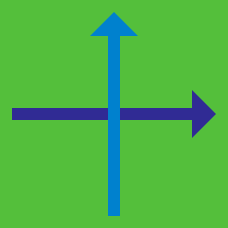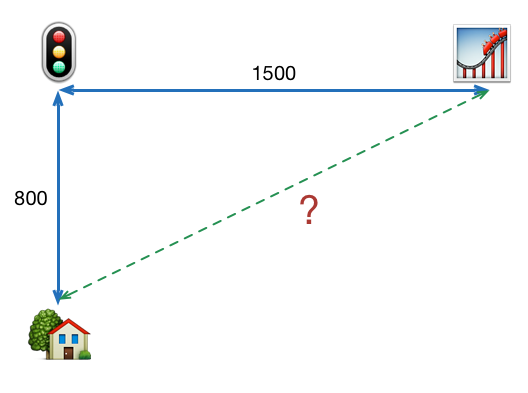Geometry

# 2D Coordinate Geometry - Problem SolvingI left my house and walked 800 meters north, before I reached the traffic intersection.
I then turned right, and walked a further 1500 meters east, to reach the amusement park.

What is the straight-line distance (in meters) from my house to the amusement park?

$a, b, c$ are real constants such that all the lines represented by $ax+by+c=0$ are concurrent at a point $P$. This point $P$ lies on the line $ax+by+c=0$ such that $7a+2b+8c=0$. What are the coordinates of $P$?

Find the equation of the line which is tangent to the circle $(x-3)^2 + (y-11)^2 = 53$ at the point $P = ( 5, 4).$

If four points on the $xy$-plane $A=(-1, a)$, $B=(b, 0)$, $C=(15, 1)$, and $D=(2, 21)$ are the vertices of parallelogram $ABCD$, what is the value of $a + b$?

Parallelogram $ABCD$ has three vertices $A=(28, 24),\ B=(3,4) \textrm{ and }\ C=(14, 36).$ If the intersection point of the two diagonals $AC$ and $BD$ is $M=(a,\ b)$, what is the value of $a + b$?

×